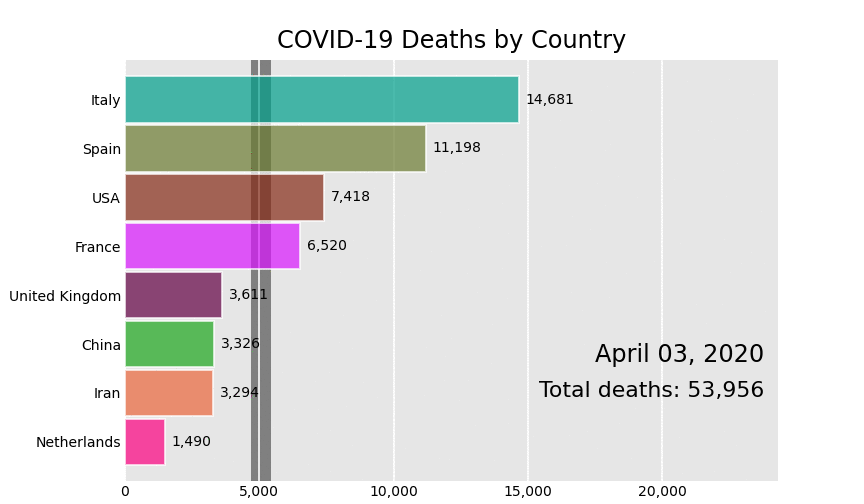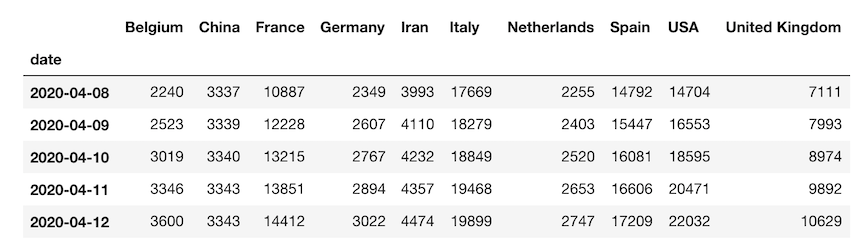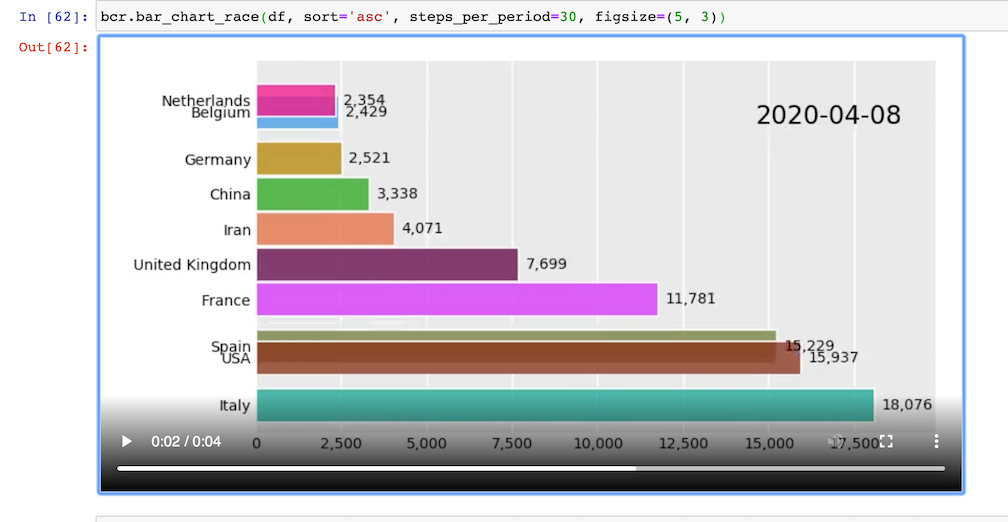Create animated bar chart races using matplotlib

# Bar Chart Race

Make animated bar chart races in Python with matplotlib.## Official Documentation

Visit the bar_chart_race official documentation for detailed usage instructions.

## Installation

Install with either:

• pip install bar_chart_race
• conda install -c conda-forge bar_chart_race

## Quickstart

Must begin with a pandas DataFrame containing 'wide' data where:

• Every row represents a single period of time
• Each column holds the value for a particular category
• The index contains the time component (optional)

The data below is an example of properly formatted data. It shows total deaths from COVID-19 for several countries by date.### Main function - bar_chart_race

There is one main function, bar_chart_race, which we use to recreate the above video. All parameters are shown with their default value except for filename and title.

import bar_chart_race as bcr
bcr.bar_chart_race(
df=df,
filename='covid19_horiz.mp4',
orientation='h',
sort='desc',
n_bars=6,
fixed_order=False,
fixed_max=True,
steps_per_period=10,
interpolate_period=False,
label_bars=True,
bar_size=.95,
period_label={'x': .99, 'y': .25, 'ha': 'right', 'va': 'center'},
period_fmt='%B %d, %Y',
period_summary_func=lambda v, r: {'x': .99, 'y': .18,
's': f'Total deaths: {v.nlargest(6).sum():,.0f}',
'ha': 'right', 'size': 8, 'family': 'Courier New'},
perpendicular_bar_func='median',
period_length=500,
figsize=(5, 3),
dpi=144,
cmap='dark12',
title='COVID-19 Deaths by Country',
title_size='',
bar_label_size=7,
tick_label_size=7,
shared_fontdict={'family' : 'Helvetica', 'color' : '.1'},
scale='linear',
writer=None,
fig=None,
bar_kwargs={'alpha': .7},
filter_column_colors=False)


### Save animation to disk or return HTML

Leave the filename parameter as None to return the animation as HTML. If you are running a Jupyter Notebook, it will automatically be embedded into it.

bcr.bar_chart_race(df=df, filename=None)### Customization

There are many options to customize the bar chart race to get the animation you desire. Below, we have an animation where the maximum x-value and order of the bars are set for the entire duration. A custom summary label and perpendicular bar of median is also added.

def period_summary(values, ranks):
top2 = values.nlargest(2)
return {'s': s, 'x': .95, 'y': .07, 'ha': 'right', 'size': 8}

bcr.bar_chart_race(df_baseball, period_length=1000,
fixed_max=True, fixed_order=True, n_bars=10,
figsize=(5, 3), period_fmt='Season {x:,.0f}',
title='Top 10 Home Run Hitters by Season Played')## Project details

This version0.1.00.0.80.0.60.0.50.0.40.0.30.0.20.0.1

Uploaded source
Uploaded py3